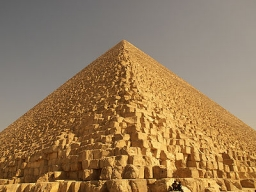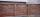# Bricks pyramid

How many 50cm x 32cm x 30cm brick needed to built a 272m x 272m x 278m pyramid?

n =  107122667

### Step-by-step explanation:Did you find an error or inaccuracy? Feel free to write us. Thank you!Tips to related online calculators
Do you want to calculate greatest common divisor two or more numbers?
Tip: Our volume units converter will help you with the conversion of volume units.
Do you want to round the number?
Do you want to perform natural numbers division - find the quotient and remainder?

#### You need to know the following knowledge to solve this word math problem:

We encourage you to watch this tutorial video on this math problem:

## Related math problems and questions:

• Brick wallGarden 70 m long and 48 m wide should surround with wall 2.1 meters high and 30 cm thick. Wall will be built on the garden ground. How many will we need bricks if to 1 m³ is required approximately 300 bricks?
• Diofant 2Is equation 70x +52y = 34 solvable on the set of integers Z?
• TissuesThe store got three kinds of tissues - 132 children, 156 women and 204 men. Tissues each species were packed into boxes after the number of pieces the same for all three types (and greatest). Determine the number, if you know that every box has more than
• Four poplarsFour poplars are growing along the way. The distances between them are 35 m, 14 m, and 91 m. At least how many poplars need to be dropped to create the same spacing between the trees? How many meters will it be?
• QuotientFind quotient before the bracket - the largest divisor 51 a + 34 b + 68 121y-99z-33
• TilesHall has dimensions 325 &time; 170 dm. What is the largest size of square tiles that can be entire hall tiled, and how many we need them?
• Reminder and quotientThere are given the number C = 281, D = 201. Find the highest natural number S so that the C:S, D:S are with the remainder of 1,
• Insulate houseThe property owner wants to insulate his house. The house has these dimensions 12, and 12 m is 15 m high. The windows have 6 with dimensions 170 and 150 cm. Entrance doors are 250 and 170 cm in size. How many square meters of polystyrene does he need?
• BricksHow many bricks are needed for a wall 14.5 m long, 2 m high, and 45 cm thick, if 285 bricks are needed for one m3 wall?
• The woodenThe wooden block measures 12 cm, 24 cm, and 30 cm. Peter wants to cut it into several identical cubes. At least how many cubes can he get?
• Cuboid wallsCalculate the cuboid volume if its different walls have an area of 195cm², 135cm², and 117cm².
• BricksOpenings in perforated bricks occupy 10% and brick has dimensions 30 cm, 15 cm and 7.5 cm. Calculate a) the weight of a perforated bricks, if you know that the density of the full brick material is p = 1800 kg/m3 (1.8 kg/dm3) b) the number of perforated
• On Children'sOn Children's Day, the organizers bought 252 chewing gums, 396 candies and 108 lollipops. They want to make as many of the same packages as possible. Advise them what to put in each package and how many packages they can make this way.
• CubesCarol with cut bar 12 cm x 12 cm x 135 cm to the cubes. Find the sum of all the surfaces of the resulting cubes.
• Bricks wallThere are 5000 bricks. How high wall thickness of 20 cm around the area which has dimensions 20 m and 15 m can use these bricks to build? Brick dimensions are 30 cm, 20 cm and 10 cm.
• PegsFrom two sticks 240 cm and 210 cm long, it is necessary to cut the longest possible pegs for flowers so that no residues remain. How many pegs will it be?
• Trees in alleyThere are four trees in the alley between which the distances are 35m, 15m and 95m. Trees must be laid in the spaces so that the distance is the same and the maximum. How many trees will they put in and what will be the distance between them?